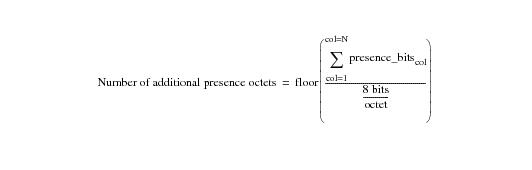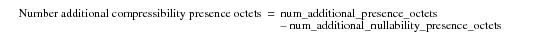# 15.00 - Presence Octets - Teradata Database

prodname
vrm_release
15.00
category
User Guide
featnum
B035-1094-015K

### Presence Octets

The number of additional presence octets for a table is a measure of how many octets (see “Presence Bits” on page 780) are required to define the multi-value compressibility of all the values for all the columns for a given table beyond those needed to express nullability. “Equation 9: Additional Presence Octets” presents the formula for calculating the number of additional presence octets in a table.

#### Equation 9: Additional Presence Octetswhere:

 This term … Specifies … floor(x) a function that returns the greatest integer less than or equal to x. This function is common in the mathematics library of most programming languages. See SQL Functions, Operators, Expressions, and Predicates for documentation of the Teradata SQL implementation of the FLOOR function. The FLOOR function rounds the result of the expression up to the nearest integer, which is the exact number of presence octets required to store the compression information for the given table. Σ presence_bitscol the sum of all the presence bits used for all columns (from column 1 through column N) in the table. 8 bits/octet 8 bits define an octet. This term produces the number of presence octets required to contain the presence bits used by all value compressed columns in the table. Because the value can be a non-integer, it must be rounded up to the next highest integer number.

You can calculate the individual contributions of additional nullability presence octets and additional compressibility presence octets to the total additional presence octets required to compress column values and nulls using “Equation 10: Additional Presence Octets for Nullability” on page 729 and “Equation 11: Additional Presence Octets for Multi-Value Compressibility” on page 730.

Note that available presence octet bits are always used exhaustively before adding additional presence octets to the row header.

#### Equation 10: Additional Presence Octets for Nullability#### Equation 11: Additional Presence Octets for Multi-Value CompressibilityThe following table provides examples of representative figures for multi-value compression.

 Number of   Presence Bits for         Nullability Additional Presence Octets   for Nullability Residual Bits in Presence  Octets After Accounting          for Nullability Number of Presence Bits      for Compressibility Additional Presence              Octets for          Compressibility 3 0 4 2 0 8 1 7 7 0 8 1 7 8 1 12 1 3 39 5 12 1 3 43 5